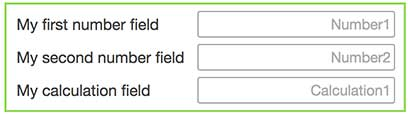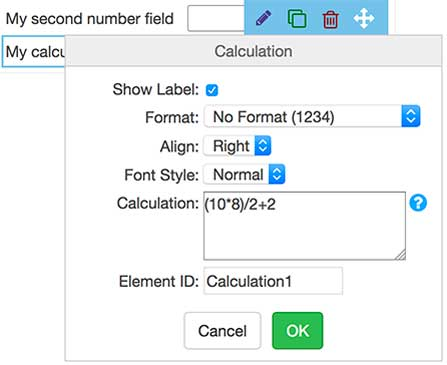One of the more advanced features of the Form Designer, is the ability to include calculations in forms.

By using the Calculation element in the Form Designer, you can perform calculations based on values in Number fields or other Calculation fields.

## Example 1

2. Click Form Designer3. Drag two Number fields to the form canvas

4. Drag a calculation field to the canvas.In the example, the text labels have been changed. Those labels have no impact.

Notice how the fields by default are named "Number1", "Number2", "Calculation1". Those can be changed by you also, and they are the variables that are used in a calculation.

5. Mouse over the calculation field, and click the pencil iconNotice that the Calculation by default is set to a formula, (10*8)/2+2. The result of the formula is 42.

If you do not know the meaning of "42", you need to read this article: Answer to the Ultimate Question of Life, the Universe, and Everything

Non-withstanding, 42 might be the calculation you are looking for.

6. Change the calculation to reference the Number fields variables, such that the calculation is the multiplication of the two numbers.6. Click OK

7. Click the Publish button. Now try the form on the iPad.

## Example 2

Expanding on example 1, the second example with perform a calculation on calculation field used in Example 1.

1. Drag in another Number field, and another calculation element2. Mouse of over Calculation2, and click the pencil icon3. Click OK

4. Click the Publish button. Now try the form on the iPad.